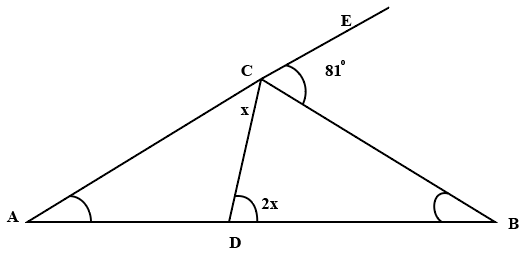Question 6

# In the figure (not drawn to scale) given below, if $$AD=CD=BC$$ and angle $$BCE = 81^\circ$$, how much is the value of angle $$DBC$$?Solution

Given,
AD = DC, this implies angle ACD = angle DAC = x
DC = BC, this implies angle CDB = angle CBD = 2x
$$\angle\ DCB$$ = 180 - x - 81 = 99 - x
In triangle BCD, sum of the angles is 180 degrees
99 - x + 2x + 2x = 180
3x = 81
x = 27
$$\angle\ DBC$$ = 2x = 54 degrees